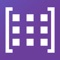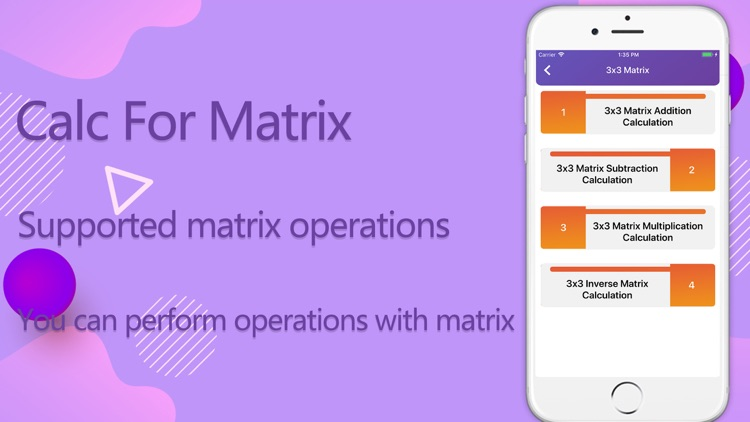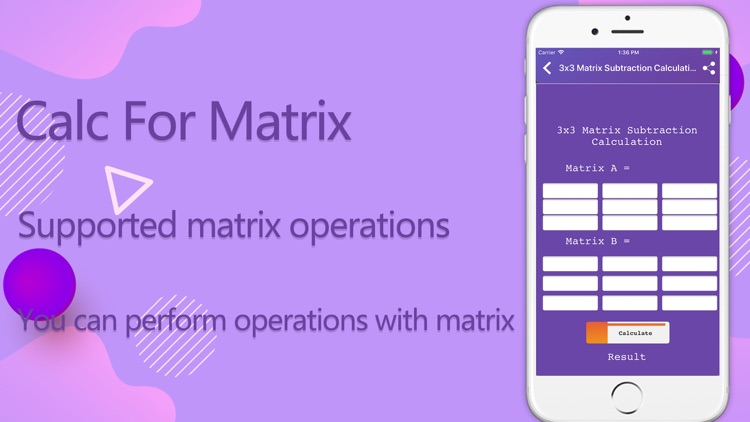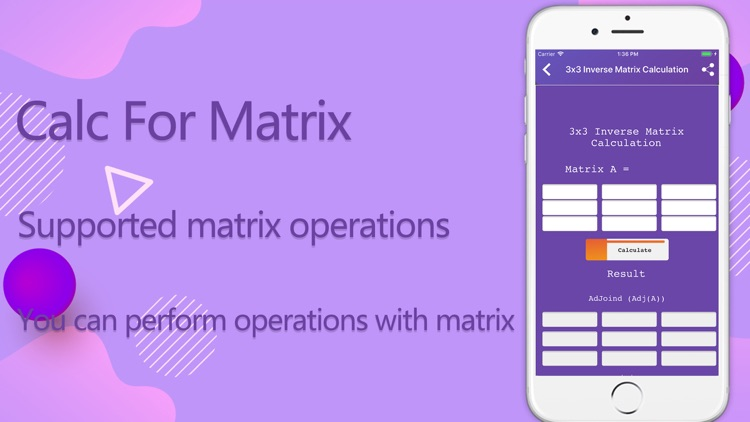## Calc For Matrix 2019# Calc For Matrix 2019

by Emily DorisCalc For Matrix 2019### App Details

Version
1.1
Rating
NA
Size
5Mb
Genre
Education Utilities
Last updated
July 23, 2019
Release date
July 20, 2019

### App Store Description

Calc For Matrix 2019

Through this application, you can perform operations with matrix

Supported matrix operations:

- 2x2 Matrix Multiplication Calculation
- 2x2 Inverse Matrix Calculation
- 2x2 Matrix Multiplication Calculation
- 3x3 Matrix Subtraction Calculation
- 3x3 Matrix Multiplication Calculation
- 3x3 Inverse Matrix Calculation
- 4x4 Matrix Addition & Subtraction Calculation
- 4x4 Matrix Multiplication Calculation
- 4x4 Inverse Matrix Calculation
- 4x4 Matrix Determinant Calculator
- Matrix Determinant Calculation
- Squared Matrix Calculation
- Transpose Matrix Calculation
- Cramer's Rule Determinant Calculation
- Matrix Gauss Elimination Calculation

If you like this app or have any feedback comment, please let us know.

Disclaimer:
AppAdvice does not own this application and only provides images and links contained in the iTunes Search API, to help our users find the best apps to download. If you are the developer of this app and would like your information removed, please send a request to [email protected] and your information will be removed.